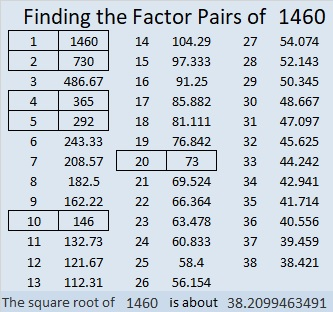# 1460 and Level 5

### Today’s Puzzle:

Can you use logic to figure out where the numbers from 1 to 10 must go so that the given clues and the factors you find make this puzzle a multiplication table?### Factors of 1460:

• 1460 is a composite number.
• Prime factorization: 1460 = 2 × 2 × 5 × 73, which can be written 1460 = 2² × 5 × 73
• 1460 has at least one exponent greater than 1 in its prime factorization so √1460 can be simplified. Taking the factor pair from the factor pair table below with the largest square number factor, we get √1460 = (√4)(√365) = 2√365
• The exponents in the prime factorization are 2, 1, and 1. Adding one to each exponent and multiplying we get (2 + 1)(1 + 1)(1 + 1) = 3 × 2 × 2 = 12. Therefore 1460 has exactly 12 factors.
• The factors of 1460 are outlined with their factor pair partners in the graphic below.As I mentioned earlier, √1460 = 2√365.
Most years have 365 days in them, but not 2020, our current year. Nevertheless,
1460 ÷ 73 × 101= 2020.

1460 is the sum of two squares in two different ways:
28² + 26² = 1460
38² + 4² =1460

1460 is the hypotenuse of FOUR Pythagorean triples:
108-1456-1460, calculated from 28² – 26², 2(28)(26), 28² + 26²
304-1428-1460, calculated from 2(38)(4), 38² – 4², 38² + 4²
876-1168-1460 which is (3-4-5) times 292
960-1100-1460 which is 20 times (48-55-73)